# 3.6   Inheritance

This section under major construction. Rename to GUI?

## Event-based programming.

Event-based programming is a powerful example that extols the virtues of callbacks. Provides more refined control over interactive methods in StdDraw. We now describe how the support event-based interactions (mouse clicks and keystrokes) within Draw.java by using the interface DrawListener.java.
 public interface DrawListener { public void mousePressed (double x, double y); public void mouseDragged (double x, double y); public void mouseReleased(double x, double y); public void mouseClicked(double x, double y); public void keyTyped(char c); public void keyPressed(int keycode); public void keyReleased(int keycode); } 
• Keyboard input. Program MovingJoker.java displays an image (the joker) and moves it when the user types one of the keys 'i', 'j', 'k', or 'l'.
• Mouse input. Program Squares.java takes a command line parameter N and draws an N-by-N grid of cells. When the user clicks in a cell, draw a blue circle. Repeat, but alternate colors between red and blue. Mouse clicks and dragging.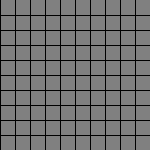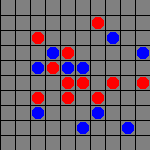Or use a hex grid and create Lite-Brite images.
• Mouse dragging. Program Graffiti.java waits for the user to click and drag the mouse, and draws a trail as the user drags the mouse. It changes colors as the user drags.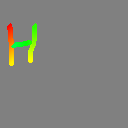## Sound filters.

Do something with sound filters, e.g., high-pass filter, echo filter, etc.

Paint program. Program Paint.java waits for the user to click and drag the mouse, and draws a circle of the user-specified diameter. It uses XOR mode to erase and redraw the circle.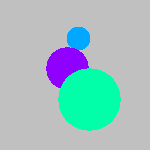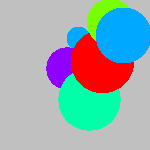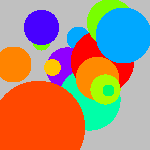Picture zoom. Zoom in or zoom out of a photo by clicking the mouse.

Linear regression. Program LeastSquares.java plots points wherever the user clicks, and then draws the least squares fit line through the points after each point is added.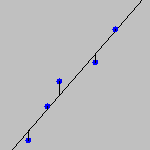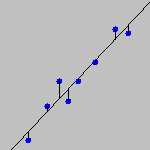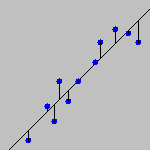## Computational geometry.

Shape super class (or perhaps an interface instead). Rectangle, circle, point, triangle, polygon subclasses.
 public abstract class Shape { private Color color; public void setColor(Color c) { color = c; } public Color getColor() { return color; } public abstract void draw(); public abstract double area(); public abstract double perimeter(); } 
Create random shapes and compute area using Monte Carlo integration.
 public static void main(String[] args) { int M = Integer.parseInt(args); // # shapes int N = Integer.parseInt(args); // # samples Shape[] shapes = new Shape[M]; for (int i = 0; i < M; i++) { int r = (int) (Math.random() * 3); if (r == 0) shapes[i] = new Rectangle(); else if (r == 1) shapes[i] = new Circle(); else shapes[i] = new Triangle(); } for (int i = 0; i < M; i++) shapes[i].draw(); for (int j = 0; j < N; j++) { Point p = new Point(); boolean contains = false; for (int i = 0; i < N; i++) { if (shapes[i].contains(p)) contains = true; } if (contains) yes++; } System.out.println("Estimate of area = " + 1.0 * yes / N); } 

### Voronoi diagram.

Program Voronoi.java plots points wherever the user clicks, and then draws the Voronoi diagram. The Voronoi diagram (and its cousin, the Delaunay triangulation) have numerous applications ranging from anthropology to typography. References: voronoi.com and David Eppstein.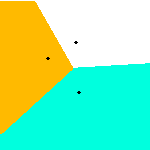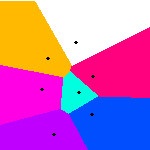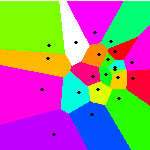### Delaunay triangulation.

Program Delaunay.java plots points wherever the user clicks, and then draws the Delaunay triangulation. The Delaunay triangulation has numerous scientific applications. Perhaps the most important is finite element methods for computational fluid dynamics. Here, a PDE is simulated over a continuous surface, which is decomposed into a mesh of triangular elements. The Delaunay triangulation has many appealing computational properties (triangles are as close to equilateral as possible), and it is efficiently computable. Note also that the boundary is the convex hull.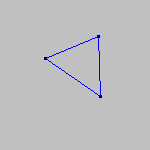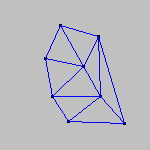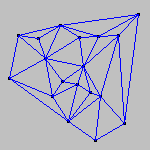Simple discretized algorithm: for each pixel look at the input point to which it is closest (ala Voronoi), and do the same for its 8 neighboring pixels. If the inputs points are different, draw a line between the two points. Include a big enclosing triangle so that it works for boundary edges.

GUI widgets. Program Celsius2Fahrenheit.java provides a text field for the user to enter a temperature in degrees Fahrenheit, and converts this to degrees Celsius. (To be replaced by an interesting example....) When the user types enter, this generates an ActionEvent and triggers a callback to the actionPerformed method.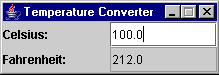Program ImageProcessor.java illustrates using a pulldown menu in an image processing application. The user can load an image from the file system in JPEG, PNG, or GIF format. Then the user can apply one of several image processing filters to the image. At any time the user can save the current image. We use the JFileChooser library for the open and save dialog boxes. This represents a significant amount of code that we don't want or need to re-implement every time we open a file. Moreover, by always using the built-in widget, we provide the user with a consistent interface. We re-use our Picture.java library for creating the image and accessing the pixels. Its method getJPanel enables us to embed the picture into the frame.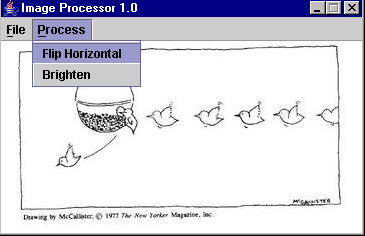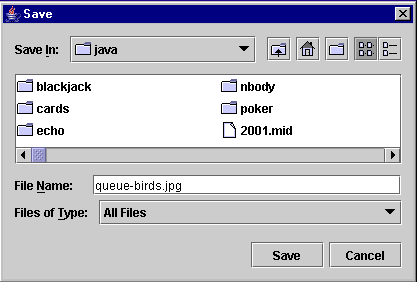Program Slider.java draws a spirograph. The figure is characterized by three parameters, R, r, and a. The user can adjust the parameter R using a slider, and the figure is drawn as the slider is moved. Program Spirograph.java is a fancier version that provides 3 sliders with labels.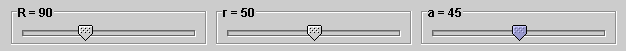### Normal approximation to binomial distribution.

Helper class LabeledSlider.java. Program Binomial.java is a visualization that illustrates the normal approximation to the binomial distribution. Relies on Draw.java and ProbStat.java.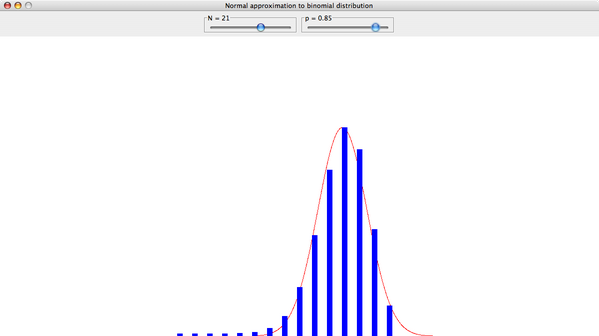GUI demo. Program GUI.java is a template of an application where the user can click buttons or click and draw points on a map. It uses the image file map.png.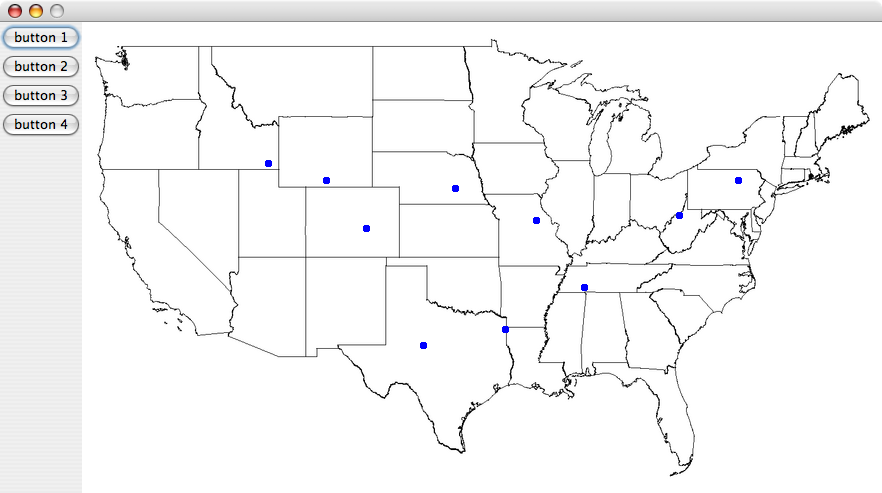BlackJack. Blackjack is a popular online card game. In a simple version (See the Exercises for more complicated variants), there are two players, the dealer and the gambler. Each player is dealt 2 cards from a standard 52 card deck of cards. The goal is to have a hand value of closer than 21 than the dealer, without going over. (Jacks, queens, and kings count as 10, aces count as 1 or 11, the cards 2 through 9 count as indicated. The suits are irrelevant.) The dealer's strategy is fixed: hit on 16 or below, stand or 17 or above. The gambler has two basic options: to draw another card (hit) or to stop (stick). The gambler is permitted to continue hitting until they exceed 21. The gambler gets to see one of the dealer's cards, and should base their hitting strategy, in part, on this information.

One of the main challenges in designing a computerized Blackjack program is the design. We embrace the OOP paradigm and decompose our program into components representing real world objects. A number of objects spring to mind: an individual playing card, a deck of cards, a player, and the game itself. Our program is organized accordingly.

• Card.java is a data type that represents one of the 52 playing cards. Each Card stores the rank and suit of a single playing card. There are many sensible ways to do this. We choose to maintain two instance variables, suit and rank. The variable suit stores an integer from 0 to 3, representing clubs, hearts, diamonds, and spades, respectively. The variable suit stores an integer from 0 to 12, representing 2, 3, 4, ..., Jack, Queen, King, and Ace. We a toString method to return a text description of the Card and a draw method to draw it using our graphics library. For Blackjack, it is also convenient to have a method isAce to tell us whether the card is an Ace, and a method rank to tell us the Blackjack value of a card. We also include an instance variable isConcealed that stores a boolean value to indicate whether or not the card is face down or face up. We use two helper methods conceal and reveal to set the isConcealed bit.
• Deck.java is a data type that represents a deck of 52 playing cards. The Deck constructor creates a new deck of 52 cards, in sorted order. It has a method shuffle for shuffling the deck, a method size for returning the number of cards left in the deck, and a method get for deleting and returning the top card. As in Section 3.2 we use the library cards.jar to display images of the playing cards.
• Player.java is a data type that represents one of the blackjack players, either the dealer or the gambler. It stores the hand of cards that a player holds. In Blackjack, a player will never have more than 9 cards, but to make the object more general, we enable each Player to hold up to 52 cards. We include a method add to insert a Card, a method reset to discard the hand, and a method value to return the blackjack value of the hand. We make Player extend TurtlePanel so that it has drawing capabilities. Perhaps, a better design would be to have separate Player and PlayerPanel objects.
• BlackJack.java represents the game itself, including the GUI. Here is a screen snapshot of the game in progress.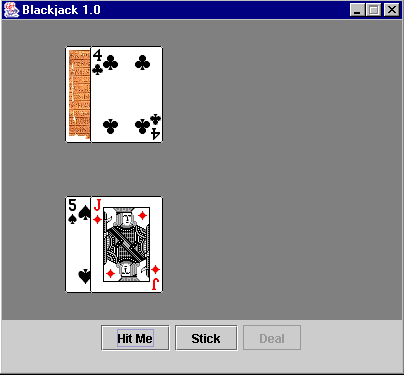#### Q + A

Q. What does a.equals(b) do if a and b are arrays?

A. Same things as (a == b). Use Arrays.equal(a, b) to check if elements within the array are equal (rather than just the array references).

#### Exercises

1. Write an interface ComplexFunction that represents a function that takes a complex input and returns a complex number.
2. Write subclasses Put and Call of Option.
3. Generalize Mandelbrot.java so that you can use it with different update formulas (not just z = z^2 + c) that the user can choose. Be careful about concluding that the point is not in the set when |z| > 2.
4. Discrete probability distributions. Create a data type DiscreteDistribution that takes an array of values (that sum to 1) as input. Preprocess (e.g., compute cumulative sums) to make it more efficient. Have methods: sample(), pdf(), cdf(), mean(), variance().
5. Continuous probability distribution. Create an interface ContinuousDistribution that supports pdf(), cdf(), sample(), inverseCdf(). Could provide reference implementation of inverseCdf() and sample() in superclass, using binary search to implement inverseCdf().
6. Write an interface for twice differentiable functions. Write a client that uses Newton's method to find the root of an arbitrary twice-differentiable function.
7. Particle. Create a data type for particle with position, velocity, mass, and charge. Create a subclass Nucleus that contains additional instance variables = number of protons, number of neutrons.
8. Molecule. Create a data type for molecules with center of mass. Create a subclass PeptideChain that has the additional method of extracting a subchain.
9. Chess pieces. Create a data type for chess pieces. Inherit from the base class Piece and create subclasses Rook, King and so on. Include a method isLegalMove(Position a, Position b) that termines whether the given piece can move from a to b.
10. Tetris pieces. Create a data type for Tetris pieces. Inherit from the base class Piece and create subclasses for each of the piece types with implementations for rotate() and drop().
11. Groups, rings, and fields. Use implementaiton inheritance to implement the following algebraic structures: ring, group, field, semigroup, abelian group, commutative ring.
12. Matrix inheritance. Use implementation inheritance to implement the following types of N-by-N matrices: matrix, symmetric matric, diagonal matrix, identity matrix, banded matrix. Include methods for solving systems of linear equations and matrix multiplication. Special the algorithm to the type of matrix.
13. Matrix inheritance. Why would the following be a bad design decision if matrices were mutable (e.g., can change entries in the matrix)?
 public class SymmetricMatrix extends Matrix { ... } 
The subclass SymmetricMatrix inherits all of the operations of Matrix, including the one to change the value of entry i-j. If the client did this without changing j-i, it would create a SymmetricMatrix object that did not have symmetric entries.
14. Shape3D. Create an interface / abstract class for 3D shapes, e.g., volume(), surfaceArea(), sample(), boundingBox3D(), sample(). Then create data types Cylinder, Sphere, Cube.
15. Circle extends Point. Analyze whether the following is an appropriate use of inheritance.
• circle extends point
• point extends circle
16. Write a program to create a resistor network for the following circuits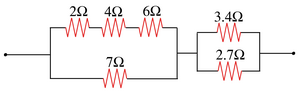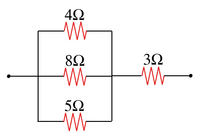17. Write a program to create a resistor network for the following circuits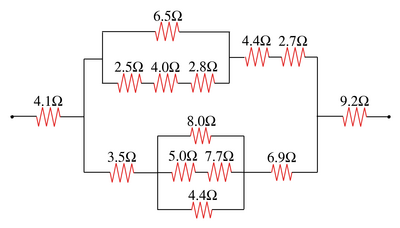18. Modify the the resistor network data type to support operations involving getting the potential and power of various components of a circuit according to Ohm's Law.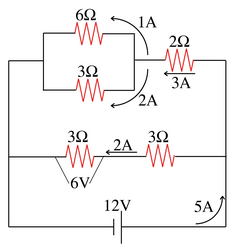19. Modify Graffiti.java so the size of the circle is adjusted if the user types the key 0-9.
20. Modify Graffiti.java so that the color of the circle changes according to the spectrum.
21. Modify Graffiti.java so that each time the user clicks, it draws a circle centered at the current location with the integer i printed in the middle for the ith circle.
22. Modify Graffiti.java so it when you click on points, it connects the new one to the previously drawn one with a line segment.
23. Modify Graffiti.java so that it saves the graffiti in a file when the user types 's'.
24. Write a program ColorMandelbrotZoom.java that plots the Mandelbrot set using a 256 color palette such as mandel.txt, and lets the user zoom in as in MandelbrotZoom.java.
25. Consider the following implementation of the compareTo() method for String. How does the third line help with efficiency?
 public int compareTo(Object x) { String s = this; String t = (String) x; if (s == t) return 0; int n = Math.min(s.length(), t.length()); for (int i = 0; i < n; i++) { if (s.charAt(i) < t.charAt(i)) return -1; else if (s.charAt(i) > t.charAt(i)) return +1; } return s.length() - t.length(); } 

Answer: avoid directly comparing all n characters if s and t are references to the same string.

#### Creative Exercises

1. Asteroids. Write a program Spaceship.java that lets the user rotate and accelerate a spaceship, as in the game of Asteroids. When the user type 'j' or 'l' the spaceship should rotate 2 degrees counterclockwise or clockwise. When the user type 'i' or 'k' the spaceship should accelerate in the current direction. Use periodic boundary conditions to ensure that the spaceship always remains visible. Use the image starfield.jpg for the background and starship.gif for the spaceship.
2. Sokoban. Implement the Japanese game of Sokoban. Use arrows keys to move the porter. Include an undo feature.
3. Capacitor networks. Write a superclass CapacitorNetwork and subclasses Capacitor, Parallel, and Series to build up a capacitor network. Works exactly like resistor networks, except rules for series and parallel and reversed.
4. Slider puzzle. Write a program that chooses 8 of the 9 images slider1.gif through slider9.gif and arranges them in a 3-by-3 grid with one blank tile blank.gif. If the user clicks a tile and it is adjacent to the empty tile, "slide" that tile to the empty square and play a sound effect.5. 15-slider puzzle. Write a program that prints 15 tiles labeled 1 through 15 in a 4-by-4 grid. If the user clicks a tile and it is adjacent to the empty tile, "slide" that tile to the empty square and play a sound effect.
6. Pong with multiples balls. Modify Pong.java so that there are three simultaneous balls.
7. Pong with spin. Modify Pong.java so that if the ball strikes the paddle while the paddle is moving, the ball spins according to the laws of physics.
8. Blackjack. Redo where the user types H for hit, S for stick, and D for deal. Use Turtle graphics.
9. Blackjack variants.
1. Use 4 decks instead of one.
2. Keep track of the number of times the player has won and lost, and print out the results after each game.
3. Add a button that allows the player to place 1, 2, or 4 dollar bets on each hand.
4. If the gambler gets 21 with two cards (blackjack), then she wins 1.5 times her bet. A natural blackjack beats a 21 obtained with three or more cards.
5. Allow the gambler to double down: if the gambler has exactly two cards, then the gambler can double the size of the bet if she agree to receive exactly one additional card.
6. Allow the gambler to buy insurance if the dealer's face up card is an ace.
7. Allow the gambler to surrender: before making any other decisions, the gambler can fold her cards, and receive half her bet back.
8. Allow the gambler to split pairs: if the gambler's two initial cards are the same (ignoring the suits), she can split the hand into two individual hands, and play them independently.
10. Blackjack strategy. Create an automated strategy for the gambler and see how well it does by playing a million hands. (You'll probably want to lose the GUI for this type of experiment.) Use the basic strategy. By counting cards, you may be able to win money consistently. If your program does so, plan a trip to Las Vegas.
11. Poker. Write a program that evaluates 5 card poker hands and prints out the best hand according to the standard order: royal flush, straight flush, four of a kind, full house, flush, straight, three of a kind, two pair, one pair, high card. If there is a tie, a pair of 8's beats a pair of 7's, etc.
12. Five of a kind. Suppose you add a joker that acts as a wild card in five card poker. Determine mathematically whether a royal flush should beat five of a kind or vice versa. Repeat if you add two jokers.
13. Texas Hold 'Em. Texas Hold 'Em is a popular variant of poker. Before each hand, the dealer shuffles a standard 52 card deck. The dealer deals two cards face down (the hole cards) to each player. Then, the dealer deals five cards face up (the communal cards) in the middle of the table. Each player selects their best 5 card hand from the hole cards and the communal cards. Write a program to determine the best hand given two hole cards and five communal cards.
14. World Series of Poker. The World Series of Poker is played using Texas Hold 'Em. There is a round of betting after the first three communal cards (the flop) are dealt, and then again after the fourth communal card (the turn card), and again after the fifth communal card (the river card). ESPN televises the event and displays the probability that each hand will win after the flop, based on knowledge of every player's hole cards and the flop. Write a program to determine the exact probabilities by enumerating over all pairs of possible turn and river cards.
15. Moving circle. Write a program that draws a circle. It moves left, right, up, or down, if the user types the corresponding key. It changes color to red, green, or blue if the user types 'r', 'g', or 'b'.
16. Coordinates. Modify Graffiti.java so that it writes the (x, y) coordinate of each mouse click to the screen, centered at the location (x, y) of the mouse click.
17. Paint. Modify Graffiti.java so that it changes the color to red, green, or blue if the user types 'r', 'g', or 'b'. Also, have it change shapes from square to circle if the user types 's' or 'c'. Change the diameter of the circle or square if the user types '1' (small) through '9' (large).
18. Concentration. Implement the game of concentration. There is an N-by-N grid of cards, displayed face down. The user selects two of them to reveal. If they match, then the two cards are removed from the grid. Otherwise, the user is charged with 1 point and the cards are concealed. Repeat until the board is cleared.
19. Minesweeper. Implement the game of Minesweeper.
20. Tic-tac-toe. Implement the game of tic-tac-toe with two human players (or one human and one computer player).

## Resistor networks.

When electricity moves through a wire, it is subject to electrical friction or resistance. When a resistor with resistance R is connected across a potential difference V, Ohm's law asserts that it draws current I = V / R and dissipates power V^2 / R. A network of resistors connected across a potential difference behaves as a single resistor, which we call the equivalent resistance. The simplest way to connect more than resistor network is to use one of the following two composition rules. Each leads to a particularly simple formula for computing the equivalent resistance of the resulting network.
• Series: Connect two resistor networks end-to-end in a single chain. If the equivalent resistance of the two networks are R1 and R2, then the equivalent resistance of the combined network is given by additive rule: R1 + R2. Ex: old Christmas lights - if one burns out, then it would break circuit and all other lights would go out.
• Parallel: Connect two resistor networks in parallel. If the equivalent resistance of the two networks are R1 and R2, then the equivalent resistance of the combined network is given by the reciprocal rule: 1 / (1/R1 + 1/R2). Parallel (e.g., electrical wiring in homes so that you can turn on lights independently).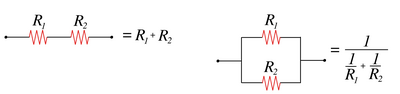Although the two rules for composition are simple, we can repeatedly apply them to design arbitrarily complex circuits. When series and parallel components are mixed in a circuit, we can simplify each series or parallel section into one resistor equivalent to its total resistance and apply this idea recursively.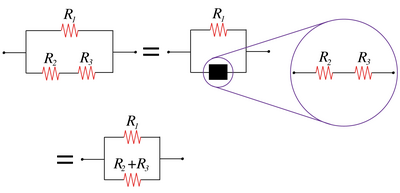The resistors R2 and R3 and connected in series, so we apply the series formula to them to get a total resistance of R2 + R3. This total resistance is shown in the black box, which is connected in parallel with resistor R1. Now apply the parallel formula to R1 and the box, obtaining the circuit's resistance, 1 / (1/R1 + 1/(R2+R3)).

However, not all resistor networks are series-parallel networks, e.g., a bridge with 5 resistors. In these cases, we need to use a more powerful technique known as Kirchoff's method (see section xyz).

To create series-parallel resistor networks in Java, we define a superclass Circuit.java that encapsulates the basic properties of a resistor network. For now, each network has a method resistance that returns the equivalent resistance of the circuit.

 public abstract class Circuit { public abstract double resistance(); } 
A series-parallel resistor network is either (i) a single resistor or (ii) built up by connecting two resistor networks in series or parallel. We create three subclasses Resistor.java, Series.java, and Parallel.java. Our goal is to be able to compose circuits as in the following code fragment, which represents the circuit depicted below.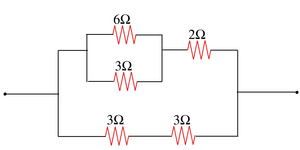Circuit a = new Resistor(3.0); Circuit b = new Resistor(3.0); Circuit c = new Resistor(6.0); Circuit d = new Resistor(3.0); Circuit e = new Resistor(2.0); Circuit f = new Series(a, b); Circuit g = new Parallel(c, d); Circuit h = new Series(g, e); Circuit circuit = new Parallel(h, f); double R = circuit.resistance(); 
• The class Resistor contains a constructor which sets the resistance in Ohms and an accessor method to return it.
 public class Resistor extends Circuit { private double resistance; public Resistor(double r) { resistance = r; } public double resistance() { return resistance; } } 
• The class Series contains a constructor which takes two resistor circuit objects as inputs and represents a circuit with the two components in series.
 public class Series extends Circuit { private Circuit a; private Circuit b; public Series(Circuit a, Circuit b) { this.a = a; this.b = b; } public double resistance() { return a.resistance() + b.resistance(); } } 
• The class Parallel is almost identical to Series except that it uses the reciprocal rule instead of the additive rule to compute the equivalent resistance.
 public double resistance() { double R1 = a.resistance(); double R2 = b.resistance(); return 1.0 / (1.0 / R1 + 1.0 / R2); } 

Electricity moving through a wire is comprised of voltage (V), current (I), and resistance (R). The resistance measures the electrical friction. Ohm's law relates the three quantities by the famous formula V = I * R. When a battery is attached to a circuit, it creates a "potential difference" across the circuit. Smaller potential differences are created across each circuit segment. The potential difference across each section depends on whether the circuit is series or parallel. For a parallel circuit, the potential difference across each branch is equal to the potential difference across the whole parallel circuit. For a series circuit, first find the current (I) from Ohm's law I = V/R, where V is the potential difference across the series circuit and R is its total resistance. The potential difference across each resistor is equal to the current times the resistance of that resistor.